Anúncio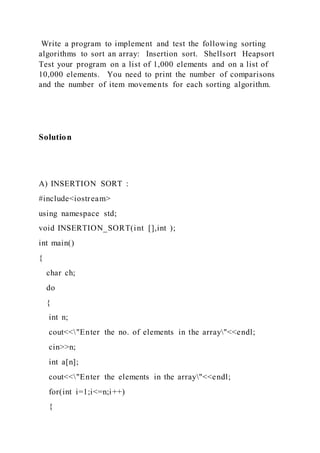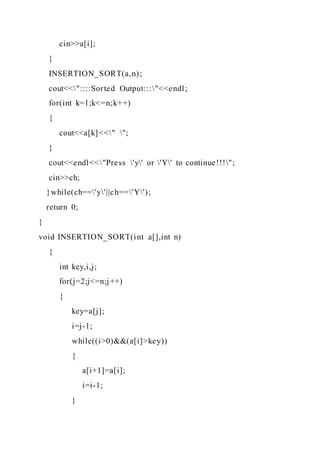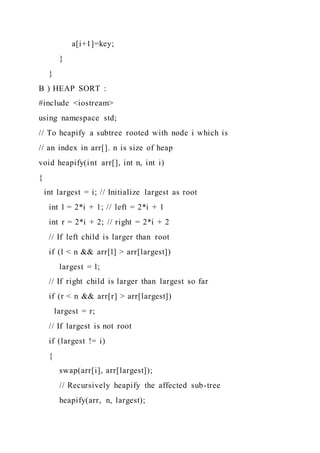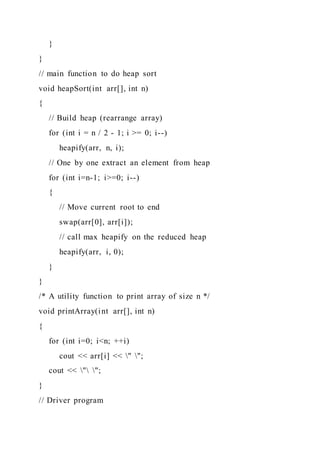Anúncio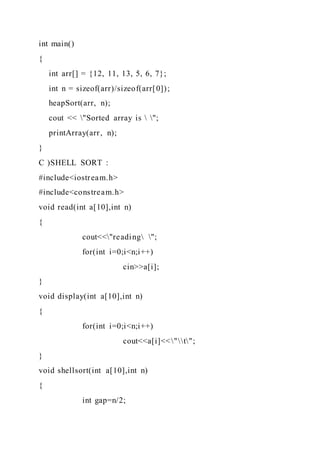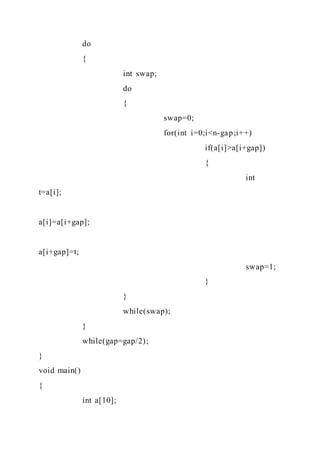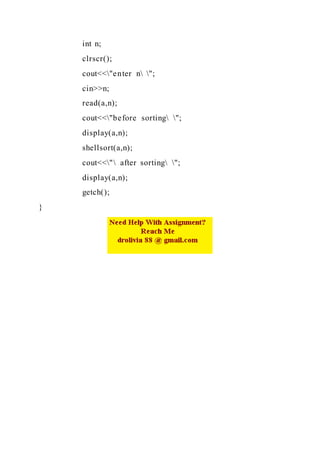Próximos SlideShares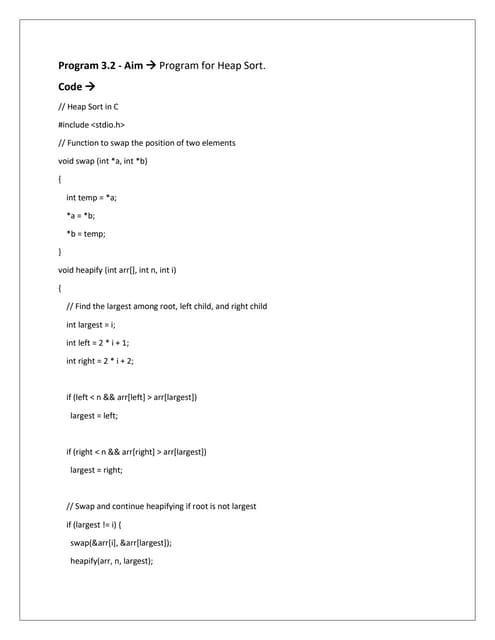DAA Lab Work.docx
Carregando em ... 3
1 de 7
Anúncio

### Write a program to implement and test the following sorting algorithm.docx

1. Write a program to implement and test the following sorting algorithms to sort an array: Insertion sort. Shellsort Heapsort Test your program on a list of 1,000 elements and on a list of 10,000 elements. You need to print the number of comparisons and the number of item movements for each sorting algorithm. Solution A) INSERTION SORT : #include<iostream> using namespace std; void INSERTION_SORT(int [],int ); int main() { char ch; do { int n; cout<<"Enter the no. of elements in the array"<<endl; cin>>n; int a[n]; cout<<"Enter the elements in the array"<<endl; for(int i=1;i<=n;i++) {
2. cin>>a[i]; } INSERTION_SORT(a,n); cout<<"::::Sorted Output:::"<<endl; for(int k=1;k<=n;k++) { cout<<a[k]<<" "; } cout<<endl<<"Press 'y' or 'Y' to continue!!!"; cin>>ch; }while(ch=='y'||ch=='Y'); return 0; } void INSERTION_SORT(int a[],int n) { int key,i,j; for(j=2;j<=n;j++) { key=a[j]; i=j-1; while((i>0)&&(a[i]>key)) { a[i+1]=a[i]; i=i-1; }
3. a[i+1]=key; } } B ) HEAP SORT : #include <iostream> using namespace std; // To heapify a subtree rooted with node i which is // an index in arr[]. n is size of heap void heapify(int arr[], int n, int i) { int largest = i; // Initialize largest as root int l = 2*i + 1; // left = 2*i + 1 int r = 2*i + 2; // right = 2*i + 2 // If left child is larger than root if (l < n && arr[l] > arr[largest]) largest = l; // If right child is larger than largest so far if (r < n && arr[r] > arr[largest]) largest = r; // If largest is not root if (largest != i) { swap(arr[i], arr[largest]); // Recursively heapify the affected sub-tree heapify(arr, n, largest);
4. } } // main function to do heap sort void heapSort(int arr[], int n) { // Build heap (rearrange array) for (int i = n / 2 - 1; i >= 0; i--) heapify(arr, n, i); // One by one extract an element from heap for (int i=n-1; i>=0; i--) { // Move current root to end swap(arr, arr[i]); // call max heapify on the reduced heap heapify(arr, i, 0); } } /* A utility function to print array of size n */ void printArray(int arr[], int n) { for (int i=0; i<n; ++i) cout << arr[i] << " "; cout << " "; } // Driver program
5. int main() { int arr[] = {12, 11, 13, 5, 6, 7}; int n = sizeof(arr)/sizeof(arr); heapSort(arr, n); cout << "Sorted array is "; printArray(arr, n); } C )SHELL SORT : #include<iostream.h> #include<constream.h> void read(int a,int n) { cout<<"reading "; for(int i=0;i<n;i++) cin>>a[i]; } void display(int a,int n) { for(int i=0;i<n;i++) cout<<a[i]<<"t"; } void shellsort(int a,int n) { int gap=n/2;
6. do { int swap; do { swap=0; for(int i=0;i<n-gap;i++) if(a[i]>a[i+gap]) { int t=a[i]; a[i]=a[i+gap]; a[i+gap]=t; swap=1; } } while(swap); } while(gap=gap/2); } void main() { int a;
7. int n; clrscr(); cout<<"enter n "; cin>>n; read(a,n); cout<<"before sorting "; display(a,n); shellsort(a,n); cout<<" after sorting "; display(a,n); getch(); }
Anúncio